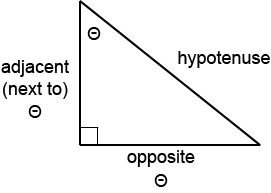# Trigonometric Functions and Right Triangles

When dealing with right triangles (triangles that have one 90 degree angle) in trigonometry, the biggest things to realize is that no matter what size the triangle is, the ratios of the lengths of the sides stay the same. So, it is very natural to give these ratios names – and that’s where the right triangle definitions of the trig functions comes from!In the triangle above, the right angle is marked with a small square. The other two angles are acute angles (have measures less than 90 degrees). Either one of these could be the angle we are interested in since the trig functions will be in terms of which side is next to (adjacent) to our angle and which side is opposite of our angle. Therefore, it is important to pay attention to which angle is of interest anytime you work with these definitions.

## Definitions

Given an angle $$\theta$$ (theta) like in the picture above, we will define the six trigonometric functions as:

$$\sin(\theta) = \dfrac{\textrm{opposite}}{\textrm{hypotenuse}}$$

$$\cos(\theta) = \dfrac{\textrm{adjacent}}{\textrm{hypotenuse}}$$

$$\tan(\theta) = \dfrac{\textrm{opposite}}{\textrm{adjacent}}$$

$$\csc(\theta) = \dfrac{\textrm{hypotenuse}}{\textrm{opposite}}$$

$$\sec(\theta) = \dfrac{\textrm{hypotenuse}}{\textrm{adjacent}}$$

$$\cot(\theta) = \dfrac{\textrm{adjacent}}{\textrm{opposite}}$$

## Applying the Definitions

Let’s see how the definitions work in an actual example.

### Example

Consider the triangle below.Find the exact values of the six trigonometric functions of the angle $$\theta$$.

### Solution

When it says “exact value”, it means that you should not give a decimal approximation. This means that once you get a simplified fraction, you will leave the answer as it is.

Looking at the triangle, the side opposite the angle theta has a length of 2 while the side adjacent has a length of 1. Further, the hypotenuse has a length of $$\sqrt{5}$$.

This is all that is needed to calculate the values of all the trig functions. So, start plugging them in and simplifying!

\begin{align}\sin(\theta) &= \dfrac{\textrm{opposite}}{\textrm{hypotenuse}}\\ &= \dfrac{2}{\sqrt{5}}\end{align}

\begin{align}\cos(\theta) &= \dfrac{\textrm{adjacent}}{\textrm{hypotenuse}}\\ &= \dfrac{1}{\sqrt{5}}\end{align}

\begin{align}\tan(\theta) &= \dfrac{\textrm{opposite}}{\textrm{adjacent}}\\ &= \dfrac{2}{1}\\ &= 2\end{align}

\begin{align}\csc(\theta) &= \dfrac{\textrm{hypotenuse}}{\textrm{opposite}}\\ &= \dfrac{\sqrt{5}}{2}\end{align}

\begin{align}\sec(\theta) &= \dfrac{\textrm{hypotenuse}}{\textrm{adjacent}}\\ &= \dfrac{\sqrt{5}}{1}\\ &= \sqrt{5}\end{align}

\begin{align}\cot(\theta) &= \dfrac{\textrm{adjacent}}{\textrm{opposite}}\\ &= \dfrac{1}{2}\end{align}

Some textbooks and classes require you to “rationalize” the denominator. This means that where ever there is a root in the denominator like $$\sin(\theta)$$ and $$\cos(\theta)$$ above, you must multiply the top and bottom of the fraction by that root in order to remove it from the denominator. Again, this isn’t always required, but if we do it here:

\begin{align}\sin(\theta) &= \dfrac{2 \times \sqrt{5}}{\sqrt{5} \times \sqrt{5}}\\ &= \dfrac{2\sqrt{5}}{5}\end{align}

\begin{align}\cos(\theta) &= \dfrac{1 \times \sqrt{5}}{\sqrt{5 \times \sqrt{5}}}\\ &= \dfrac{\sqrt{5}}{5}\end{align}

Important! – These rationalized answers are mathematically the same as the answers above them. Its just a different way of writing the answers.

Now, what if you are only given two of the lengths in the triangle? Does this mean we can’t find the other lengths? Not at all. In fact, you only need two of any of the lengths as you can see in the following example.

### Example

A right triangle has a hypotenuse of length 10 and a leg of length 6. Find the exact values of the six trigonometric functions of the angle $$\theta$$ if $$\theta$$ is adjacent to the leg of length 6.

### Solution

To be able to find these values, the first thing you will need to do is figure out what the side length is for that missing leg. What is helpful here is the Pythagorean Theorem. It says that if $$a$$ and $$b$$ are the legs of a right triangle and if $$c$$ is the hypotenuse, then:

$$a^2 + b^2 = c^2$$

Using that here, one of the legs is 6, so say $$a = 6$$ and $$c = 10$$ since $$c$$ is the hypotenuse. Therefore:

\begin{align}6^2 + b^2 &= 10^2\\ 36 + b^2 &= 100\\ b^2 &= 100 – 36 = 64\\b &= \sqrt{64}\\ &= 8\end{align}

Now remembering that the problem said, “$$\theta$$ is adjacent to the leg of length 6”. This means that you can now draw your triangle:Using this:

\begin{align} \sin(\theta) &= \dfrac{\textrm{opposite}}{\textrm{hypotenuse}} \\ &= \dfrac{8}{10}\\ &= \dfrac{4}{5}\end{align}

\begin{align}\cos(\theta) &= \dfrac{\textrm{adjacent}}{\textrm{hypotenuse}}\\ &= \dfrac{6}{10}\\ &= \dfrac{3}{5}\end{align}

\begin{align} \tan(\theta) &= \dfrac{\textrm{opposite}}{\textrm{adjacent}}\\ &= \dfrac{8}{6}\\ &= \dfrac{4}{3}\end{align}

\begin{align}\csc(\theta) &= \dfrac{\textrm{hypotenuse}}{\textrm{opposite}}\\ &= \dfrac{10}{8}\\ &= \dfrac{5}{4}\end{align}

\begin{align}\sec(\theta) &= \dfrac{\textrm{hypotenuse}}{\textrm{adjacent}}\\ &= \dfrac{10}{6}\\ &= \dfrac{5}{3}\end{align}

\begin{align}\cot(\theta) &= \dfrac{\textrm{adjacent}}{\textrm{opposite}}\\ &= \dfrac{6}{8}\\ &= \dfrac{3}{4}\end{align}

As you can see, getting a correct picture was a big part of solving this problem. Make sure to read questions carefully to determine which values represent the different parts of the triangle and so you can determine which angle is the angle the problem is asking about.